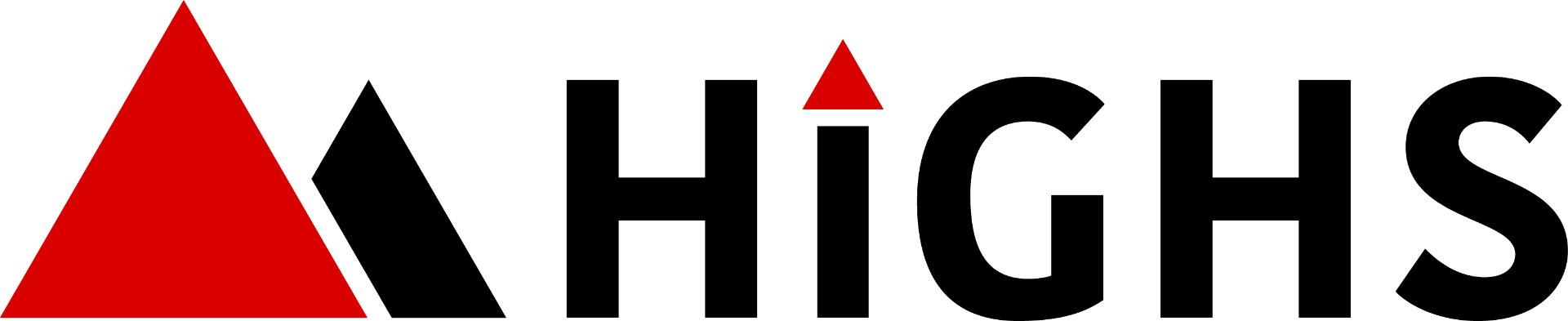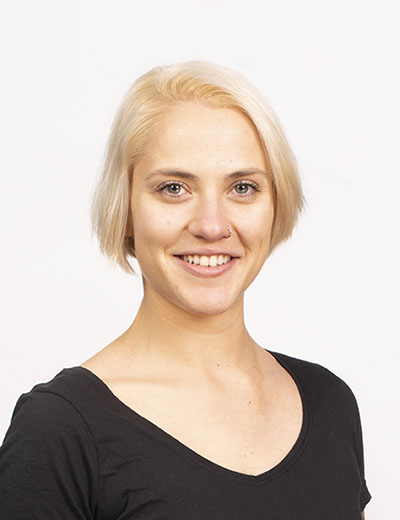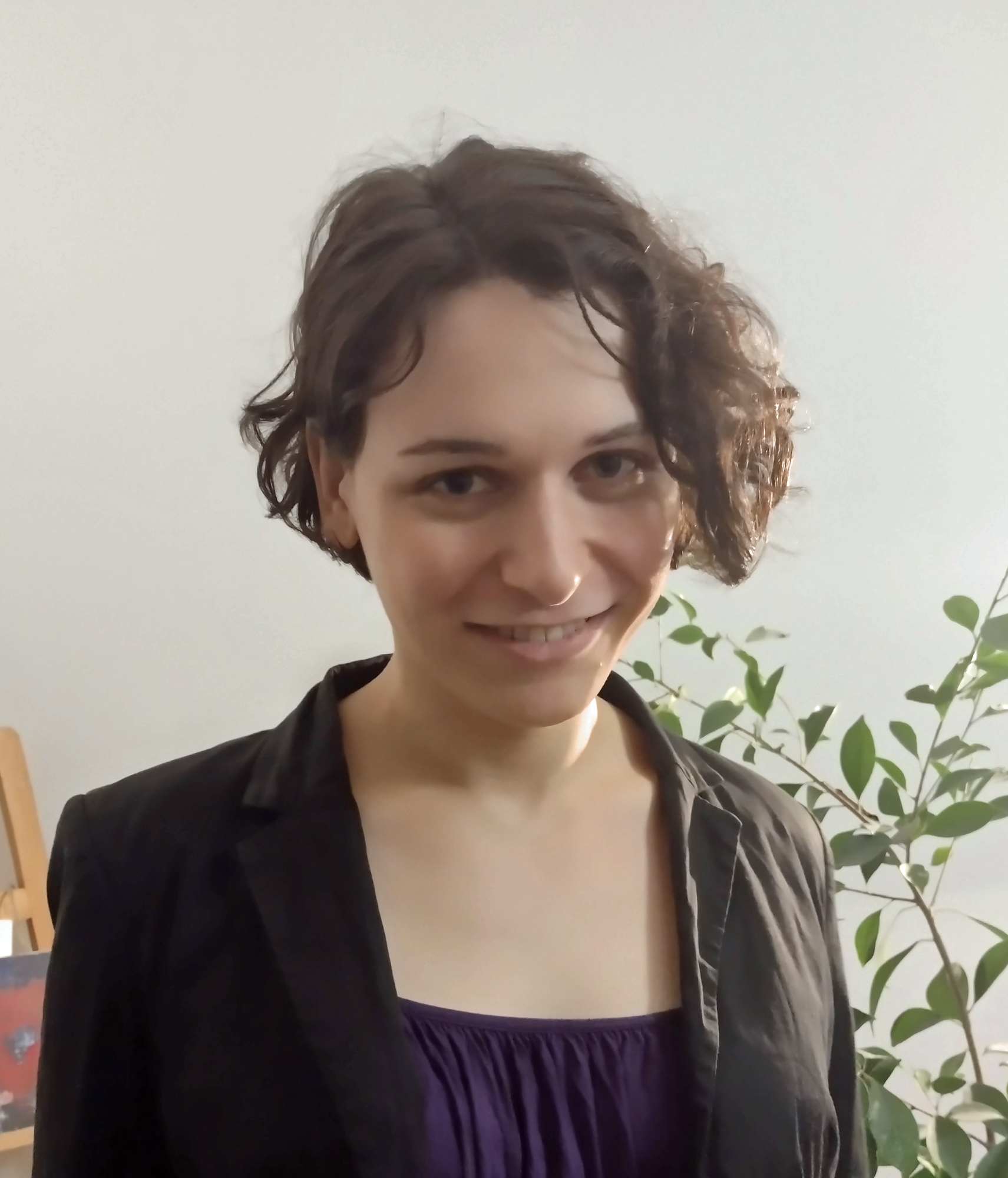## HiGHS - high performance software for linear optimization

Open source serial and parallel solvers for large-scale
sparse linear programming (LP),
mixed-integer programming (MIP), and quadratic programming (QP) models

## Get started

HiGHS is high performance serial and parallel software for solving large-scale sparse linear programming (LP), mixed-integer programming (MIP) and quadratic programming (QP) models, developed in C++11, with interfaces to C, C#, FORTRAN, Julia and Python.

HiGHS is freely available under the MIT licence, and is downloaded from Github. Installing HiGHS from source code requires CMake minimum version 3.15, but no other third-party utilities. HiGHS can be used as a stand-alone executable on Windows, Linux and MacOS. There is a C++11 library which can be used within a C++ project or, via one of the interfaces, to a project written in other languages.

Your comments or specific questions on HiGHS would be greatly appreciated, so please send an email to highsopt@gmail.com to get in touch with the team.## Documentation

Information on how to set up and use HiGHS is given in the HiGHS Documentation page.

## Background

### Authorship

HiGHS is based on the high performance dual revised simplex implementation (HSOL) and its parallel variant (PAMI) developed by Qi Huangfu. Features such as presolve, crash and advanced basis start have been added by Julian Hall and Ivet Galabova. The QP solver and original language interfaces were written by Michael Feldmeier. Leona Gottwald wrote the MIP solver. The software engineering of HiGHS was developed by Ivet Galabova.

### Citation

In the absence of a release paper, academic users of HiGHS are kindly requested to cite the following article

Parallelizing the dual revised simplex method, Q. Huangfu and J. A. J. Hall, Mathematical Programming Computation, 10 (1), 119-142, 2018. DOI: 10.1007/s12532-017-0130-5

### Terminology

Any linear optimization problem will have decision variables, a linear or quadratic objective function, and linear constraints and bounds on the values of the decision variables. A mixed-integer optimization problem will require some or all of the decision variables to take integer values. The problem may require the objective function to be maximized or minimized whilst satisfying the constraints and bounds. By default, HiGHS minimizes the objective function.

The bounds on a decision variable are the least and greatest values that it may take, and infinite bounds can be specified. A linear objective function is given by a set of coefficients, one for each decision variable, and its value is the sum of products of coefficients and values of decision variables. The objective coefficients are often referred to as costs, and some may be zero. When a problem has been solved, the optimal values of the decision variables are referred to as the (primal) solution.

Linear constraints require linear functions of decision variables to lie between bounds, and infinite bounds can be specified. If the bounds are equal, then the constraint is an equation. If the bounds are both finite, then the constraint is said to be boxed or two-sided.

The coefficients of the linear constraints are naturally viewed as rows of a matrix. The constraint coefficients associated with a particular decision variable form a column of the constraint matrix. Hence constraints are sometimes referred to as rows, and decision variables as columns. Constraint matrix coefficients may be zero. Indeed, for large practical problems it is typical for most of the coefficients to be zero. When this property can be exploited to computational advantage, the matrix is said to be sparse. When the constraint matrix is not sparse, the solution of large problems is normally intractable computationally.

It is possible to define a set of constraints and bounds that cannot be satisfied, in which case the problem is said to be infeasible. Conversely, it is possible that the value of the objective function can be improved without bound whilst satisfying the constraints and bounds, in which case the problem is said to be unbounded. If a problem is neither infeasible, nor unbounded, it has an optimal solution. The optimal objective function value for a linear optimization problem may be achieved at more than point, in which case the optimal solution is said to be non-unique.

When none of the decision variables is required to take integer values, the problem is said to be continuous. For continuous problems, each variable and constraint has an associated dual variable. The values of the dual variables constitute the dual solution, and it is for this reason that the term primal solution is used to distinguish the optimal values of the decision variables. At the optimal solution of a continuous problem, some of the decision variables and values of constraint functions will be equal to their lower or upper bounds. Such a bound is said to be active. If a variable or constraint is at a bound, its corresponding dual solution value will generally be non-zero: when at a lower bound the dual value will be non-negative; when at an upper bound the dual value will be non-positive. When maximizing the objective the required signs of the dual values are reversed. Due to their economic interpretation, the dual values associated with constraints are often referred to as shadow prices or fair prices. Mathematically, the dual values associated with variables are often referred to as reduced costs, and the dual values associated with constraints are often referred to as Lagrange multipliers.

Analysis of the change in optimal objective value of a continuous linear optimization problem as the cost coefficients and bounds are changed is referred to in HiGHS as ranging. For an active bound, the corresponding dual value gives the change in the objective if that bound is increased or decreased. This level of analysis is often referred to as sensitivity. In general, the change in the objective is only known for a limited range of values for the active bound. HiGHS will return the limits of these bound ranges ranges, the objective value at both limits and the index of a variable or constraint that will acquire an active bound at both limits. For each variable with an active bound, the solution will remain optimal for a range of values of its cost coefficient. HiGHS will return the values of these cost ranges. For a variable or constraint whose value is not at a bound, HiGHS will return the range of values that the variable or constraint can take, the objective values at the limits of the range, and the index of a variable or constraint with a bound that will become in active at both limits.

## The Team